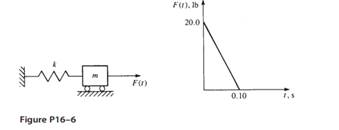### Create an Account

Home / Questions / For the spring-mass system shown in Figure P16–6 determine the mass displacement velocity ...

# For the spring-mass system shown in Figure P16–6 determine the mass displacement velocity and acceleration for five time steps using a the central difference method

For the spring-mass system shown in Figure P16–6, determine the mass displacement, velocity, and acceleration for five time steps using (a) the central difference method, (b) Newmark’s time integration method, and (c) Wilson’s method. Let k = 1200 lb/ft and m = 2 slugs.Aug 01 2020 View more View LessSubscribe To Get Solution